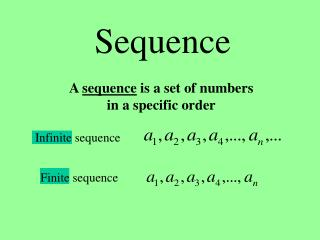Download PresentationSequence

# Sequence - PowerPoint PPT PresentationDownload Presentation## Sequence

- - - - - - - - - - - - - - - - - - - - - - - - - - - E N D - - - - - - - - - - - - - - - - - - - - - - - - - - -
##### Presentation Transcript

1. Sequence A sequence is a set of numbers in a specific order Infinite sequence Finite sequence

2. Sequences – sets of numbers

3. Ex.1 Find the first four terms of the sequence First term Second term Third term Fourth term

4. Writing Rules for Sequences We can calculate as many terms as we want as long as we know the rule or equation for an. Example: 3, 5, 7, 9, ___ , ___,……. _____ . an = 2n + 1

5. Series – the sum of a certain number of terms of a sequence Stop Formula Start “Add up the terms in the sequence beginning at term number 1 and going through term number “n”.

6. Examples

7. Infinite Sequence Infinite Series Finite Series or nth Partial Sum

8. SUMMATION NOTATION Sum of the terms of a finite sequence Upper limit of summation (Ending point) Lower limit of summation (Starting point)

9. Sequence • There are 2 types of Sequences Arithmetic: You add a common difference each time. Geometric: You multiply a common ratio each time.

10. Arithmetic Sequences Example: • {2, 5, 8, 11, 14, ...}Add 3 each time • {0, 4, 8, 12, 16, ...}Add 4 each time • {2, -1, -4, -7, -10, ...}Add –3 each time

11. Arithmetic Sequences • Find the 7th term of the sequence: 2,5,8,… Determine the pattern: Add 3 (known as the common difference) Write the new sequence: 2,5,8,11,14,17,20 So the 7th number, or a7, is 20

12. Arithmetic Sequences • When you want to find a large sequence, this process is long and there is great room for error. • To find the 20th, 45th, etc. term use the following formula: an = a1  + (n - 1)d

13. Arithmetic Sequences an = a1  + (n - 1)d Where: a1 is the first number in the sequence n is the number of the term you are looking for d is the common difference an is the value of the term you are looking for

14. Arithmetic Sequences • Find the 15th term of the sequence: 34, 23, 12,… Using the formula an = a1  + (n - 1)d, a1 = 34 d = -11 n = 15 an = 34 + (n-1)(-11) = -11n + 45 a15 = -11(15) + 45 a15 = -120

15. Arithmetic Sequences Melanie is starting to train for a swim meet. She begins by swimming 5 laps per day for a week. Each week she plans to increase her number of daily laps by 2. How many laps per day will she swim during the 15th week of training?

16. Arithmetic Sequences • What do you know? an = a1  + (n - 1)d a1= 5 d= 2 n= 15 t15 = ?

17. Arithmetic Sequences • tn = t1  + (n - 1)d • tn = 5  + (n - 1)2 • tn = 2n  + 3 • t15 = 2(15) + 3 • t15 = 33 During the 15th week she will swim 33 laps per day.

18. Arithmetic Series • The sum of the terms in a sequence are called a series. • There are two methods used to find arithmetic series: Formula Sigma Notation

19. Geometric Sequences • In geometric sequences, you multiply by a common ratio each time. • 1, 2, 4, 8, 16, ... multiply by 2 • 27, 9, 3, 1, 1/3, ...Divide by 3 which means multiply by 1/3

20. Geometric Sequences • Find the 8th term of the sequence: 2,6,18,… Determine the pattern: Multiply by 3 (known as the common ratio) Write the new sequence: 2,6,18,54,162,486,1458,4374 So the 8th term is 4374.

21. Geometric Sequences • Again, use a formula to find large numbers. • an = a1 • (r)n-1

22. Geometric Sequences • Find the 10th term of the sequence : 4,8,16,… an = a1 • (r)n-1 • a1 = 4 • r = 2 • n = 10

23. Geometric Sequences an = a1 • (r)n-1 a10 = 4 • (2)10-1 a10 = 4• (2)9 a10 = 4• 512 a10 = 2048

24. Geometric Sequences • Find the ninth term of a sequence if a3 = 63 and r = -3 a1= ? n= 9 r = -3 a9 = ? There are 2 unknowns so you must…

25. Geometric Sequences • First find t1. • Use the sequences formula substituting t3 in for tn.  a3 = 63 • a3 = a1 • (-3)3-1 • 63 = a1 • (-3)2 • 63= a1 • 9 • 7 = a1